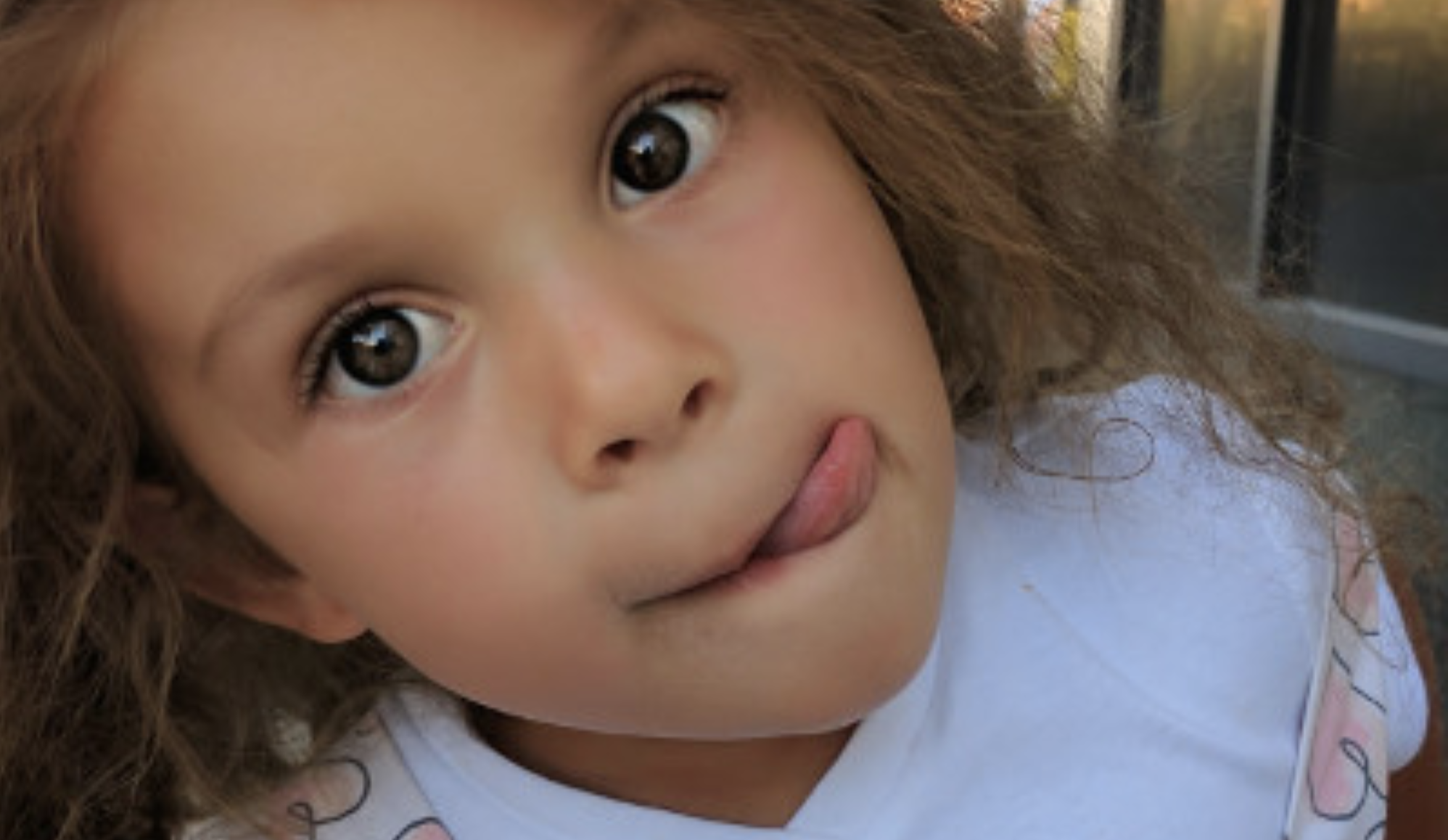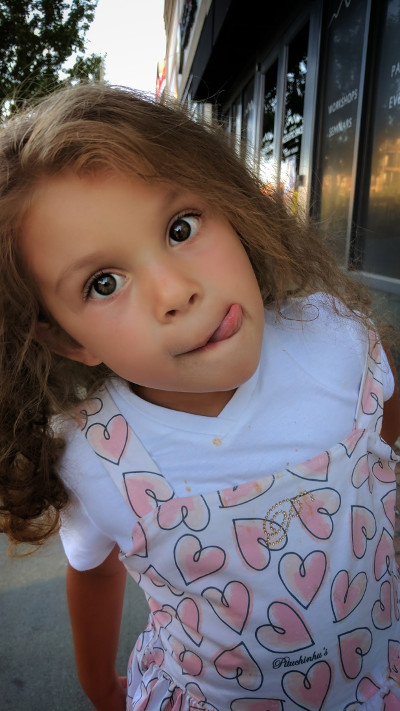# Rodrigo Silveira

## Machine Learning Painting with Linear RegressionThis is an old demo I made in 2017.

An interactive demo of using linear regression to minimize a function that “learns” what the values of a matrix of pixels should be so that it forms a photograph. This work was very early practice as I was learning the foundations of supervised learning.

As mentioned in other posts… last night (November 4, 2020) I was showing my 7 year old daughter what Twitter is. I decided to show her some of the weird stuff I’d posted over the years. One of the posts was a link to an old demo I had posted on an old version of this blog. I clicked on the link, only to realize I’d removed all my old blog posts not related to my current pursuit of AI and Machine Learning. My daughter told me that I “should never delete stuff, because then people would never know what I had to say or show them.”

That 7 year old wisdom was enough motivation for me to dig through my Github account and find the code for that and other demos, which is what you’ll find below.

# The original content

This is my first implementation of a machine learning algorithm in JavaScript. I think linear regression is an appropriate place to start, as the only thing required to get something going is to implement gradient descent. Although the code is not optimized for performance, it demonstrates the underlying concepts of minimizing a cost function in order to learn the parameters that best fit the training data.

## Demo: Learning an input image

This toy application feeds the pixel data for some input image to a linear regression model, which eventually learns what RGB value corresponds with each pixel coordinate. A more technical explanation follows.

## How linear regression “learns”

The first image in the demo above is used as the target that the algorithm is to learn. Since the goal is for the algorithm to learn every pixel in the image, I create a model with `n + 1` weights, where `n = width * height` pixels in the input image, and the additional value acts as a bias value.

The training data consists the coordinate of each pixel, with the expected output as the hex value of the corresponding pixel. Since this is a linear model (as opposed to a neural network), I’m not sure how I can use two features (such as [x, y] values) and affect as many weights as I’d like. Thus, each training sample is a vector of length `n` with all zeros, and a one representing the pixel location in question.

``````// Suppose the target image is a 2x2 image
const trainingX = [
[1, 0, 0, 0],
[0, 1, 0, 0],
[0, 0, 1, 0],
[0, 0, 0, 1],
];

const trainingY = [
[0xfff],
[0xf00],
[0x0f0],
[0x00f],
];
``````

In the example above, `trainingX` refers to pixel [0, 0], which maps to the expected value `trainingY`, which happens to be a white color.

To keep things simple, I run gradient descent for a small amount of epochs, then use the model to redraw the image pixel by pixel. The process repeats until the cost function returns below some arbitrary threshold.## JavaScript implementation of linear regression

Checkout the entire source for this at https://github.com/formigone/machine-learning/blob/master/lin-reg/LinearRegressionModel.js. My purpose with that repository is to learn and experiment, and not to produce a high performance machine learning library.

The main class is `LinearRegressionModel`, which has a simple interface:

``````/**
*
* @param {Array<Array<number>>} samples List of samples
* @param {Array<Array<number>>} labels List of vectors
*/
LinearRegressionModel.prototype.train = function(samples, labels){};

/**
*
* @param {Array<number>} inputs
*/
LinearRegressionModel.prototype.score = function(inputs){};

/**
*
* @returm {Array<number>}
*/
LinearRegressionModel.prototype.getParams = function(){};

/**
*
* @param {Array<number>} params
*/
LinearRegressionModel.prototype.setParams = function(params){};
``````

To me the most exciting part about it is the implementation of the training algorithm, which I was pleasantly surprised with how simple it was to get right within 15 minutes. Ignoring some of the logging stuff in that method, the steps are:

• Add a constant input value of 1 to each sample (to reconcile the bias weight).
• Score each sample using the current weights in the model.
• Calculate the error for each sample (`score - expected`).
• Create a new weights vector by updating each weight with `weight[y] - learningRate * sum(errors[i], samples[i])`

``````/**
*
* @param {Array<Array<number>>} samples List of samples
* @param {Array<Array<number>>} labels List of vectors
* @param {Object=} config - { learningRate, maxCost, epochs, logCost, logCallback }
*/
LinearRegressionModel.prototype.train = function (samples, labels, config) {
var maxEpochs = config.epochs || 10;
var epoch = 0;
var maxCost = config.maxCost || 0.05;
var learningRate = config.learningRate || 0.05;
var logCost = config.logCost || 100;
var logCallback = config.logCallback || function () {};
var M = samples.length;

var lr = learningRate / M;
var costFrac = 1 / (2 * M);

samples = samples.map(sample => .concat(sample));

while (epoch++ < maxEpochs) {
var scores = samples.map(sample => this.score(sample, true));

if (logCost > 0 && epoch % logCost === 1) {
var errorSquared = scores.reduce(function (acc, score, i) {
var diff = score - labels[i];
return acc + diff * diff;
}, 0);
var cost = costFrac * errorSquared;
if (Number.isNaN(cost)) {
throw new Error('Cost exploded');
}

if (cost < maxCost) {
break;
}

logCallback({ model: this, cost, epoch });
}

var errors = scores.map((score, i) => score - labels[i]);
this.params = this.params.map(function (param, col) {
return param - lr * errors.reduce((acc, error, row) => {
return acc + error * samples[row][col];
}, 0);
});
}
};
``````

My next goal is to implement stochastic gradient descent and see for myself what the trade-offs are. Next I plan on writing a simple neural network and try the same exercise. Finally, I will implement the same concept using TensorFlow and get more involved with it.

One question I’m trying to find a definitive answer to is: using linear regression, is it possible to input only two values (namely, [x, y]) and train the model to correctly output the value for all pixels colors of the image represented by the training data?

## Next post

### Machine Learning - Linear Classifier

An interactive demo showing linear regression fitting a very separable dataset. This work was very early practice as I was learning the foundations of supervised learning.